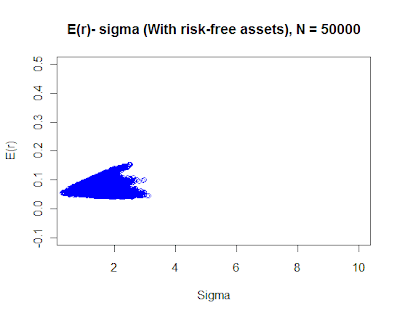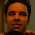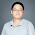## Thursday, 3 November 2011

### Modern Portfolio Optimization Theory: The idea

We were recently given a lecture (by Dr. Susan Thomas) on Harry Markowitz portfolio optimization theory, and I was really fascinating with the noble laureate's story of how he found it difficult to convince his guide about the importance of his thesis work. Little did anyone know that his thesis would get him the most respected award in academia 35 years down the lane. Let me try and illustrate what was the basic idea behind the modern portfolio theory in simple English language. The principal task of any financial advisory firm/individual is to try and solve the capital allocation problem of an agent, meaning how much money should be invested in what asset/security to get the desired rate of return with minimum risk. Lets try and visualize this problem of capital allocation from the individuals point of view. Suppose I want a 15% p.a rate of return on my investment and there is a security in the market that gives you an expected 15% annual rate of return with some risk(sigma or standard deviation) associated with that security/asset. One way for me to achieve the desired expected return is to invest in this security take the risk(sigma) associated with this security and hope that I am awarded for the risk I took and get a 15% return in the end. However, the beauty of financial markets is that since there are so many assets/securities being traded in the market, it is possible for me to diversify my investment, in simple words, park fraction of my money with different assets in a way that gives me approximately ~ 15% return but with substantially less risk. This is referred to as "diversification" in Finance parlance. The mathematics behind the results can be easily shown, one can find the maths in the lecture slides here. Let me try and illustrate this result through empirical simulation using R.

Suppose you are in a universe of only risky assets, i.e all assets that have a positive sigma. Then what Harry Markowitz did, with mathematics, was to compute the set of Expected returns that you could achieve with minimum risk (sigma). To illustrate this result I took stock returns for these 7 random companies for about 251 days, computed their respective expected returns, and the variance-co-variance matrix of the returns. With this I have all the necessary fodder to compute the E(r) and sigma for all the possible combination of weights that I can assign between these securities, meaning all possible different combinations of parking my money among these securities.

# Map your working directory using setwd()

mu = colMeans(r1[,-7])  ## Calculate the column means and storing in "mu", I have removed the 7th column from the data frame as I have a risk free asset's return there, I will come back to this later in the post.

bigsig = cov(r1[,-7]) ## Variance-co-variance matrix

m = nrow(bigsig)-1
w = diff(c(0,sort(runif(m)), 1)); ## Assigning random weights between 0-1 to "w" which will have the dimension = no. of securities between which you have to divide your investment.

rb = sum(w*mu); ## Creating matrix "rb" which stores the E(r)

sb = sum(w*bigsig*w); ## Creating matrix "sb" which stores sigma's

N = 2000  ## Number of different combinations of "w" you want to look at

## Simulating the different combinations of weights "w's" and storing the E(r) and sigma^2

for (j in 2:N) {
w = diff(c(0,sort(runif(m)), 1));
r = sum(w*mu); rb = rbind(rb,r);
s = sum(w*bigsig*w); sb = rbind(sb,s); ## Note this is sigma^2 (variance)
}

d = data.frame(rb, sb); ## Merge all the E(r) and sigmas in one data.frame.

d\$sb = sqrt(d\$sb); ## Square root the variance to get the sigmas

plot(d\$sb, d\$rb, ylab="E(r)", xlab="Sigma", col="blue",  xlim = c(0.5,10), ylim = c(-0.1,0.5), main = "E(r)- sigma (With risk free asset), N = 200000")With only Risky assets

I did this simulation for 200000 different values of weights too, for those who are curious the plot can be obtained from here.

If we close the envelope of the above mentioned points with a smooth curve (from the left hand side) we get the efficient portfolio frontier (EFF). So what the graph suggests is that one would want to choose the weights (allocations) that lie, more specifically, on the above half of the curve. It makes intuitive sense too, why would one take on more risk to get a lesser return (which is implied by the lower half of the curve). Also another observation that we can make from the above plot is that for every additional unit of returns we will have to take on an increasingly higher risk (illustrated by the upper half of the plot). This risk-return trade off graph changes its characteristics when a risk free assets comes into the picture. Lets see what happens is we incorporate the risk free asset in our data frame. Note that a risk free assets could be thought of as a government security, whose risk (sigma) is 0, hence even its co-variances with the other risky assets would be 0. The only addition to the above codes would be to replace r1[,-7] with just r1 that is include the risk free asset too.With risk-free asset

Simulation with N = 200000 here.

Look what happened to our risk-return trade off relationship, its linear!! So its a straight line instead of a smooth curve. Meaning for every additional unit of return you will have to take a fixed additional amount of risk, which will be given by the slope of the above half of the graph. This result, which sounds to be a trivial finding, can be extended to the entire universe of assets and one can compute the optimal portfolio that will give you a desired rate of return with minimum sigma. However the implementation of this methodology in the actual world is the real challenge. Computation of E(r), sigma, getting the up to date data for all the securities or assets in the universe are some of the challenges that one would face. But nevertheless it is inspiring to see how Harry Markowitz stuck to his idea and triumphed in the end with this path breaking research that shaped the financial advisory industry as we see it now.

P.S In case you want to replicate the exercise the data can be downloaded from here.

1.I find it to be a very succinct and clear review of the fundamentals.
Thanks for the excellent post.

~
ut

2.Thanks for the encouragement Bro :-)

3.Hi Thanks for the post, very insightful.

Could you explain how would you get the necessary weights to achieve a determined level of E(r) and Sigma?

E.g.: If I want a portfolio with 15% E(r) and 10% sigma (let's suppose this lies on the frontier), how do I see the actual asset allocation?

Also, is there a way to see all the asset allocation for all different efficient portfolios (according to different risks of risk and return?)

Thanks a lot
Paolo

4.Anyone still have the data? It has been removed from dropbox

5.Anyone still have the data? It has been removed from dropbox....